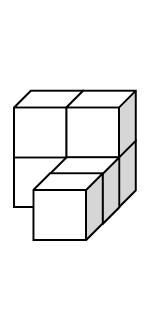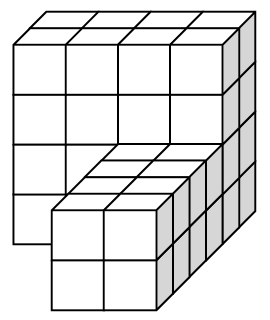### Home > INT2 > Chapter 11 > Lesson 11.1.3 > Problem11-30

11-30.

Consider the two similar solids at right.Refresh your memory about linear scale factors by re-reading the Math Notes box in Lesson 2.1.2 and in Lesson 11.1.3.

For help finding the surface area and volume of a solid, see the Math Notes box in Lesson 10.3.1.

1. What is the linear scale factor between the two solids?

$2$

2. What is the surface area of each solid? What is the ratio of the surface areas? How is this ratio related to the linear
scale factor?

The surface area can be found by counting or calculating the number of unit cube faces visible from all sides.

Surface areas are $24$ units$^²$ and $96$ units$^²$.
The ratio is $4$. It is the square of the linear scale factor.

3. Now calculate the volume of each solid. How are the volumes related? Compare this to the linear scale factor and record your observations.

The volumes can be found by counting the number of unit cubes in each solid or by finding the volume of the two rectangular prisms which make up each solid.

The volumes are $6$ units$^³$ and $48$ units$^³$.
The ratio is $8, 2^³$.